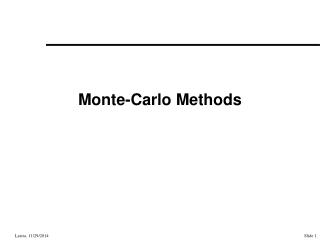DownloadDownload PresentationMonte-Carlo Methods

# Monte-Carlo Methods

Télécharger la présentation## Monte-Carlo Methods

- - - - - - - - - - - - - - - - - - - - - - - - - - - E N D - - - - - - - - - - - - - - - - - - - - - - - - - - -
##### Presentation Transcript

1. Monte-Carlo Methods

2. Topics • Kajiya’s paper • Showed that existing rendering methods are approximations of rendering equation. • Introduced path tracing. • More recent work – Lafortune, Veach.

3. Kajiya’s Rendering Equation • Expressed as point-to-point transfer • Integral is over S, union of all surfaces

4. Rendering Equation Terms • is unoccluded two-point transport intensity • Energy per unit time per unit area of source per unit area of target

5. Rendering Equation Terms • is geometry term • 0 if x not visible from x’, 1/r2if visible.

6. Rendering Equation Terms • is unoccluded emittance term

7. Rendering Equation Terms • is unoccluded three-point transport reflectance (scattering term) • Intensity scattered to x by x’ originating from x”

8. Why express as point-to-point? • Wants to set up for a path tracing solution • Point-to-point transfer of energy

9. Relationship to OtherRendering Methods • Compared rendering eqn. to conventional polygon rendering, ray tracing and distributed ray tracing. • Let scattering,

10. Relationship to “Utah Approx.” • Geometry term, g, only computed to eye. • g is ambient term. • Scattering operator, M, only operates on point sources (0), ignores visibility to light (no shadows). • M is now only sum over lights, not integration • Later extensions for shadows and area lights

11. Relationship to Ray Tracing • M0 now one reflection, one refraction, and cosine for diffuse term • Computes visibility, g, of point lights (generates shadow) • M still small sum

12. Relationship to Distributed Ray Tracing • Similar equation to Whitted’s • Now need to evaluate M as integral • M now distribution around reflection, refraction, and shadow ray • Ambient term still “elusive”

13. Relationship to Radiosity • Solves energy balance, but only for diffuse. • Derives equations for radiosity from the one presented. • Points out that solving visibility is expensive and that you may not need radiosity for all surfaces (on other hand, you might).

14. Kajiya’s Path Tracing

15. Method • At each hit, • One ray cast based on specular, diffuse, and transmission coefficients • One random ray per light • Constant number of rays per pixel (40)

16. Markov Chains for Solution p = 0.11 p = 0.08 p = 1 p = 0.02 Absorbing state

17. Algorithm • Choose pt. x’ visible from eye • Add in radiated intensity • For length of Markov path • Select pt. x” and compute g(x’, x”) • Calculate reflectance (x, x’, x”), multiply by (x’, x”) • Add contribution to pixel

18. Sampling • Most important factor in Monte Carlo • Need to avoid bias • But also need to make most out of few rays • Otherwise, noisy images • Kajiya discusses several ways. • Better to look in more modern reference • Glassner • Recent dissertations

19. Path Tracing • Postulates that even for ray tracing, following one path (probabilistically) is better. • Why? Most contribution from first ray. • Need to be careful about proportion of reflection, refraction, and shadow rays.

20. Results 256 x 256 image Ray Traced (no ambient) Path Traced Light scattered by sphere 401 minutes 533 minutes

21. Results Objects are gray, except for spheres and base. Color bleeding Caustics

22. Current Methods • Bi-directional path tracing (Lafortune and Veach) • Metropolis (Veach)

23. Pure Path Tracing

24. Pure Path Tracing Best for big luminaires. If lights small, few hits and large variance.

25. With Shadow Ray to Lights

26. With Shadow Ray to Lights Small lights OK. Best for specular surfaces.

27. Light Tracing

28. Light Tracing Small lights OK. Best for caustics.

29. Bi-Directional Path Tracing

30. Bi-Directional Path Tracing

31. Generating Samples • Generated as groups • Prefix from light joined with suffix from (to) eye (if edge is not obstructed) • Russian roulette to cut off path • Contribution must be multiplied by probability of generating path

32. Results (from Veach)

33. Metropolis • Method for importance sampling • A path is a sequence of points from a light to the eye. • Let be the image contribution function, a measure of contribution over path • is flux contributed by paths D • Strategy: generate sequence of paths with probability proportional to ƒ

34. Mutations • New path Xi+1 mutated from Xi • Probability of rejecting each mutation keeps paths distributed according to contribution • Discard start-up to reduce bias • Can be run from a set of seed paths • Run some bi-directional paths to find good seeds

35. Implementation • Path selection important • minimize rejected paths, but not too correlated • finds sub-paths and replace • or perturb vertices of path (for caustics, etc) • Mutation of “lens” path, (L|D)DS*E, to cover image pixels • Adds direct lighting • rejects path if found by Metropolis

36. Results Light for this example comes only through crack in doorway

37. Results There are specific mutations to capture caustics.

38. Advantages • Works well for difficult lighting • because it “stays” in important area • Like bi-directional path tracing there’s just a little work to get a new path

39. References • Kajiya, Jim, “The Rendering Equation”, SIGGRAPH ‘86. • Lafortune papers and thesis • Veach papers and thesis • Jensen papers • Shirley draft of MC book on his web page • Kalos & Whitlock, Monte Carlo Methods, 1986.